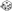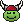Make a Random AD&D Character - Page 4 - Myth-Weavers

 Notices Hey there folks, we've made an adjustment to the rules concerning opinion pieces. You love something? Awesome! You hate something? Perfectly fine as well. You are more than welcome to express either concerning a topic in a respectful manner. We have seen an increase lately though in long rants. Be it new game systems or the end of a massive franchise story... There is no need for a massive, multi-paragraph post when the only purpose is to monopolize the conversation or start an argument. If you've already expressed your extreme dissatisfaction/devotion with [insert topic here] in a thread, you are not required to make certain everyone is informed if a new thread starts a couple months later. It is alright to move on and let it go. -MW Staff

Non Sequitur

A place for light-hearted forum games and other threads that don't promote discussion.

I'm feeling kinda lucky today...

 Race Class Ability Scores Dice Roll: 1d6z d6 Results: 6(6) Dice Roll: 1d9z d9 Results: 8(8) 1 = Human 2 = Elf 3 = Dwarf 4 = Halfling 5 = Gnome 6 = Half-Elf 1 = Fighter 2 = Ranger 3 = Paladin 4 = Cleric 5 = Druid 6 = Thief 7 = Bard 8 = Mage 9 = Illusionist Dice Roll: 3d6z d6 Results: 5, 3, 2 (Total = 10)Strength (10) Dice Roll: 3d6z d6 Results: 6, 5, 4 (Total = 15)Dexterity (15) Dice Roll: 3d6z d6 Results: 5, 3, 6 (Total = 14)Constitution (14) Dice Roll: 3d6z d6 Results: 1, 3, 2 (Total = 6)Intelligence (6) Dice Roll: 3d6z d6 Results: 1, 6, 1 (Total = 8)Wisdom (8) Dice Roll: 3d6z d6 Results: 3, 3, 4 (Total = 10)Charisma (10)

Then again, maybe not. A Half-Elf Mage...with a 6 INT.

I have to warn you, I'm feeling lucky today!

 Race Class Ability Scores Dice Roll: 1d1+6z d1 Results: 1 (Total = 7)(7) Dice Roll: 1d1+9z d1 Results: 1 (Total = 10)(10) 1 = Human 2 = Elf 3 = Dwarf 4 = Halfling 5 = Gnome 6 = Half-Elf 7 = Demi-God 1 = Fighter 2 = Ranger 3 = Paladin 4 = Cleric 5 = Druid 6 = Thief 7 = Bard 8 = Mage 9 = Illusionist 10 = Ranger/Paladin/Bard/Cleric/Druid/Mage/Illusionist Dice Roll: 18d1z d1 Results: 1, 1, 1, 1, 1, 1, 1, 1, 1, 1, 1, 1, 1, 1, 1, 1, 1, 1 (Total = 18)Strength (18) Dice Roll: 18d1z d1 Results: 1, 1, 1, 1, 1, 1, 1, 1, 1, 1, 1, 1, 1, 1, 1, 1, 1, 1 (Total = 18)Dexterity (18) Dice Roll: 18d1z d1 Results: 1, 1, 1, 1, 1, 1, 1, 1, 1, 1, 1, 1, 1, 1, 1, 1, 1, 1 (Total = 18)Constitution (18) Dice Roll: 18d1z d1 Results: 1, 1, 1, 1, 1, 1, 1, 1, 1, 1, 1, 1, 1, 1, 1, 1, 1, 1 (Total = 18)Intelligence (18) Dice Roll: 18d1z d1 Results: 1, 1, 1, 1, 1, 1, 1, 1, 1, 1, 1, 1, 1, 1, 1, 1, 1, 1 (Total = 18)Wisdom (18) Dice Roll: 18d1z d1 Results: 1, 1, 1, 1, 1, 1, 1, 1, 1, 1, 1, 1, 1, 1, 1, 1, 1, 1 (Total = 18)Charisma (18)

Nailed it! Imagine the odds!

Character: Cheaty MacFudgeliar - Strong, brilliant, handsome, nimble, wise, and hale, Cheaty breaks all the rules.Ok, ok...I'll give it another try...

 Race Class Ability Scores Dice Roll: 1d6z d6 Results: 5(5) Dice Roll: 1d9z d9 Results: 8(8) 1 = Human 2 = Elf 3 = Dwarf 4 = Halfling 5 = Gnome 6 = Half-Elf 1 = Fighter 2 = Ranger 3 = Paladin 4 = Cleric 5 = Druid 6 = Thief 7 = Bard 8 = Mage 9 = Illusionist Dice Roll: 3d6z d6 Results: 5, 3, 2 (Total = 10)Strength (10) Dice Roll: 3d6z d6 Results: 4, 5, 1 (Total = 10)Dexterity (10) Dice Roll: 3d6z d6 Results: 4, 6, 3 (Total = 13)Constitution (13) Dice Roll: 3d6z d6 Results: 3, 4, 4 (Total = 11)Intelligence (11) Dice Roll: 3d6z d6 Results: 4, 4, 2 (Total = 10)Wisdom (10) Dice Roll: 3d6z d6 Results: 3, 2, 5 (Total = 10)Charisma (10)

Character: Gnome Mage, Mediokyr MacDeadaverage - This mage dresses in gray has no particular interests, and was a solid C student at wizard school.

 Race Class Ability Scores Dice Roll: 1d6z d6 Results: 2(2) Dice Roll: 1d9z d9 Results: 5(5) 1 = Human 2 = Elf 3 = Dwarf 4 = Halfling 5 = Gnome 6 = Half-Elf 1 = Fighter 2 = Ranger 3 = Paladin 4 = Cleric 5 = Druid 6 = Thief 7 = Bard 8 = Mage 9 = Illusionist Dice Roll: 3d6z d6 Results: 6, 1, 3 (Total = 10)Strength (10) Dice Roll: 3d6z d6 Results: 1, 6, 1 (Total = 8)Dexterity (8) Dice Roll: 3d6z d6 Results: 3, 1, 1 (Total = 5)Constitution (5) Dice Roll: 3d6z d6 Results: 1, 3, 5 (Total = 9)Intelligence (9) Dice Roll: 3d6z d6 Results: 4, 1, 5 (Total = 10)Wisdom (10) Dice Roll: 3d6z d6 Results: 6, 3, 4 (Total = 13)Charisma (13)

An Elf druid who is apparently made of porcelain.

 Race Class Ability Scores Dice Roll: 1d6z d6 Results: 3(3) Dice Roll: 1d9z d9 Results: 9(9) 1 = Human 2 = Elf 3 = Dwarf 4 = Halfling 5 = Gnome 6 = Half-Elf 1 = Fighter 2 = Ranger 3 = Paladin 4 = Cleric 5 = Druid 6 = Thief 7 = Bard 8 = Mage 9 = Illusionist Dice Roll: 3d6z d6 Results: 5, 6, 3 (Total = 14)Strength (14) Dice Roll: 3d6z d6 Results: 5, 3, 4 (Total = 12)Dexterity (12) Dice Roll: 3d6z d6 Results: 3, 2, 4 (Total = 9)Constitution (9) Dice Roll: 3d6z d6 Results: 5, 4, 3 (Total = 12)Intelligence (12) Dice Roll: 3d6z d6 Results: 5, 1, 3 (Total = 9)Wisdom (9) Dice Roll: 3d6z d6 Results: 4, 5, 4 (Total = 13)Charisma (13)

Hmm... a strong dwarf illusionist...

Stupid truth, resisting simplicity.

Well. In spite of my first set actually being *playable*, let's see what I get to play next... after the inevitable TPK because none of my other party members could survive more than 2 combat rounds.

 Race Class Ability Scores Dice Roll: 1d6z d6 Results: 1(1) Dice Roll: 1d9z d9 Results: 4(4) 1 = Human 2 = Elf 3 = Dwarf 4 = Halfling 5 = Gnome 6 = Half-Elf 1 = Fighter 2 = Ranger 3 = Paladin 4 = Cleric 5 = Druid 6 = Thief 7 = Bard 8 = Mage 9 = Illusionist Dice Roll: 3d6z d6 Results: 3, 1, 2 (Total = 6)Strength (6) Dice Roll: 3d6z d6 Results: 6, 5, 6 (Total = 17)Dexterity (17) Dice Roll: 3d6z d6 Results: 6, 5, 1 (Total = 12)Constitution (12) Dice Roll: 3d6z d6 Results: 4, 4, 4 (Total = 12)Intelligence (12) Dice Roll: 3d6z d6 Results: 2, 2, 5 (Total = 9)Wisdom (9) Dice Roll: 3d6z d6 Results: 1, 5, 6 (Total = 12)Charisma (12)

 Race Class Ability Scores Dice Roll: 1d6z d6 Results: 3(3) Dice Roll: 1d9z d9 Results: 8(8) 1 = Human 2 = Elf 3 = Dwarf 4 = Halfling 5 = Gnome 6 = Half-Elf 1 = Fighter 2 = Ranger 3 = Paladin 4 = Cleric 5 = Druid 6 = Thief 7 = Bard 8 = Mage 9 = Illusionist Dice Roll: 3d6z d6 Results: 2, 5, 4 (Total = 11)Strength (11) Dice Roll: 3d6z d6 Results: 3, 6, 3 (Total = 12)Dexterity (12) Dice Roll: 3d6z d6 Results: 1, 6, 6 (Total = 13)Constitution (13) Dice Roll: 3d6z d6 Results: 4, 4, 2 (Total = 10)Intelligence (10) Dice Roll: 3d6z d6 Results: 4, 2, 4 (Total = 10)Wisdom (10) Dice Roll: 3d6z d6 Results: 6, 3, 4 (Total = 13)Charisma (13)

Let's see what happens this time.

Here we go again!
 Race Class Ability Scores Dice Roll: 1d6z d6 Results: 6(6) Dice Roll: 1d9z d9 Results: 5(5) 1 = Human 2 = Elf 3 = Dwarf 4 = Halfling 5 = Gnome 6 = Half-Elf 1 = Fighter 2 = Ranger 3 = Paladin 4 = Cleric 5 = Druid 6 = Thief 7 = Bard 8 = Mage 9 = Illusionist Dice Roll: 3d6z d6 Results: 5, 3, 3 (Total = 11)Strength (11) Dice Roll: 3d6z d6 Results: 6, 6, 1 (Total = 13)Dexterity (13) Dice Roll: 3d6z d6 Results: 1, 3, 1 (Total = 5)Constitution (5) Dice Roll: 3d6z d6 Results: 1, 5, 5 (Total = 11)Intelligence (11) Dice Roll: 3d6z d6 Results: 1, 1, 4 (Total = 6)Wisdom (6) Dice Roll: 3d6z d6 Results: 6, 3, 6 (Total = 15)Charisma (15)

Failure, Half-elf druid with 6 Wis.

Take 2!

 Race Class Ability Scores Dice Roll: 1d6z d6 Results: 1(1) Dice Roll: 1d9z d9 Results: 5(5) 1 = Human 2 = Elf 3 = Dwarf 4 = Halfling 5 = Gnome 6 = Half-Elf 1 = Fighter 2 = Ranger 3 = Paladin 4 = Cleric 5 = Druid 6 = Thief 7 = Bard 8 = Mage 9 = Illusionist Dice Roll: 3d6z d6 Results: 5, 6, 4 (Total = 15)Strength (15) Dice Roll: 3d6z d6 Results: 4, 1, 3 (Total = 8)Dexterity (8) Dice Roll: 3d6z d6 Results: 6, 5, 2 (Total = 13)Constitution (13) Dice Roll: 3d6z d6 Results: 3, 3, 6 (Total = 12)Intelligence (12) Dice Roll: 3d6z d6 Results: 2, 5, 6 (Total = 13)Wisdom (13) Dice Roll: 3d6z d6 Results: 1, 6, 5 (Total = 12)Charisma (12)

 Race Class Ability Scores Dice Roll: 1d6z d6 Results: 3(3) Dice Roll: 1d9z d9 Results: 9(9) 1 = Human 2 = Elf 3 = Dwarf 4 = Halfling 5 = Gnome 6 = Half-Elf 1 = Fighter 2 = Ranger 3 = Paladin 4 = Cleric 5 = Druid 6 = Thief 7 = Bard 8 = Mage 9 = Illusionist Dice Roll: 3d6z d6 Results: 4, 4, 3 (Total = 11)Strength (11) Dice Roll: 3d6z d6 Results: 6, 3, 6 (Total = 15)Dexterity (15) Dice Roll: 3d6z d6 Results: 6, 6, 5 (Total = 17)Constitution (17) Dice Roll: 3d6z d6 Results: 2, 5, 4 (Total = 11)Intelligence (11) Dice Roll: 3d6z d6 Results: 6, 3, 6 (Total = 15)Wisdom (15) Dice Roll: 3d6z d6 Results: 4, 2, 1 (Total = 7)Charisma (7)

A dwarf illusionist...very tough and dexterous, wise but far from being charming.

 Race Class Ability Scores Dice Roll: 1d6z d6 Results: 4(4) Dice Roll: 1d9z d9 Results: 6(6) 1 = Human 2 = Elf 3 = Dwarf 4 = Halfling 5 = Gnome 6 = Half-Elf 1 = Fighter 2 = Ranger 3 = Paladin 4 = Cleric 5 = Druid 6 = Thief 7 = Bard 8 = Mage 9 = Illusionist Dice Roll: 3d6z d6 Results: 3, 2, 2 (Total = 7)Strength (7) Dice Roll: 3d6z d6 Results: 1, 3, 1 (Total = 5)Dexterity (5) Dice Roll: 3d6z d6 Results: 3, 4, 4 (Total = 11)Constitution (11) Dice Roll: 3d6z d6 Results: 6, 6, 6 (Total = 18)Intelligence (18) Dice Roll: 3d6z d6 Results: 5, 5, 3 (Total = 13)Wisdom (13) Dice Roll: 3d6z d6 Results: 1, 1, 4 (Total = 6)Charisma (6)

Molin the halfling thief, barely able to lift a sword or bow, but he sure does get through locks quick.